Số lượng: 0

Tổng cộng: 0,00

0

# Cube

### Cube

This animation demonstrates the components (vertices, edges, diagonals and faces) of the cube, one of the Platonic solids.

Toán học

Từ khoá

khối lập phương, đỉnh, cạnh lề, đối mặt, mặt chéo, không gian chéo, khuôn mặt hàng xóm, hình học không gian, toán học

Các mục liên quan

### Các cảnh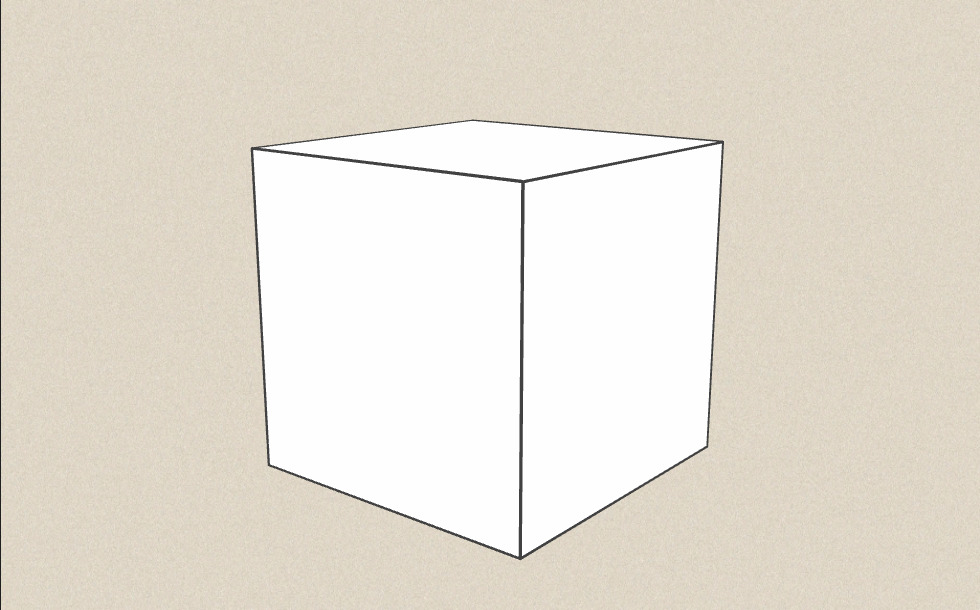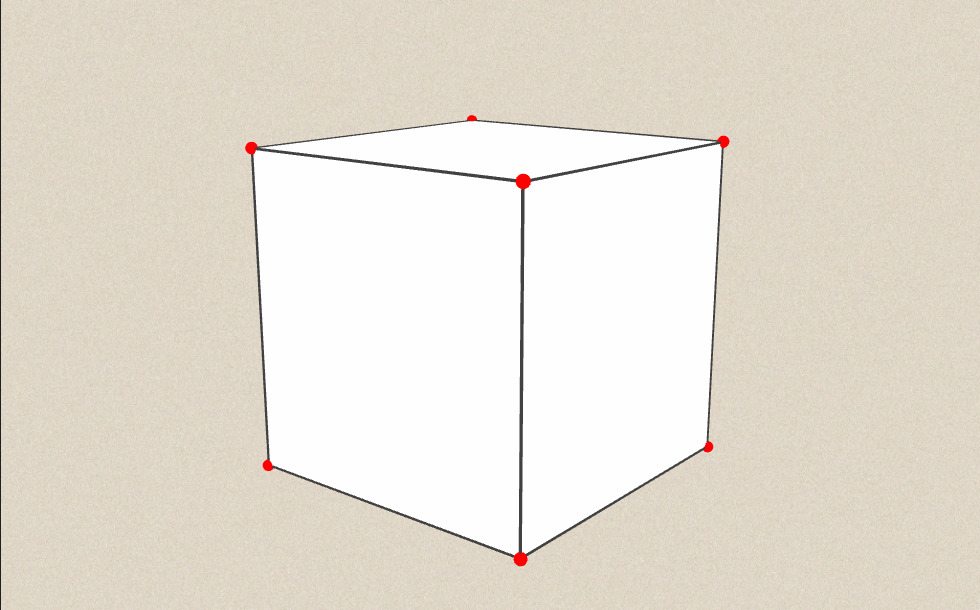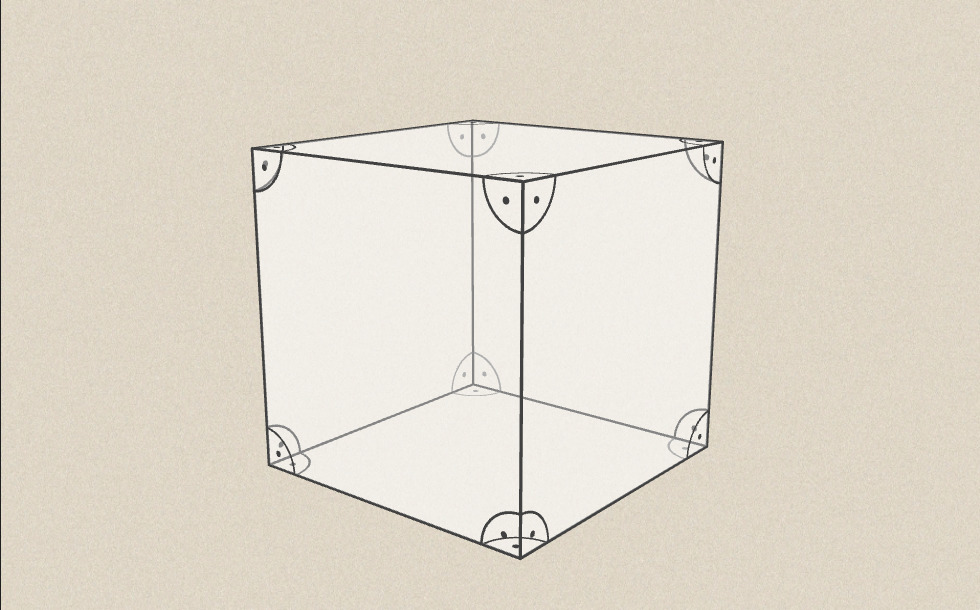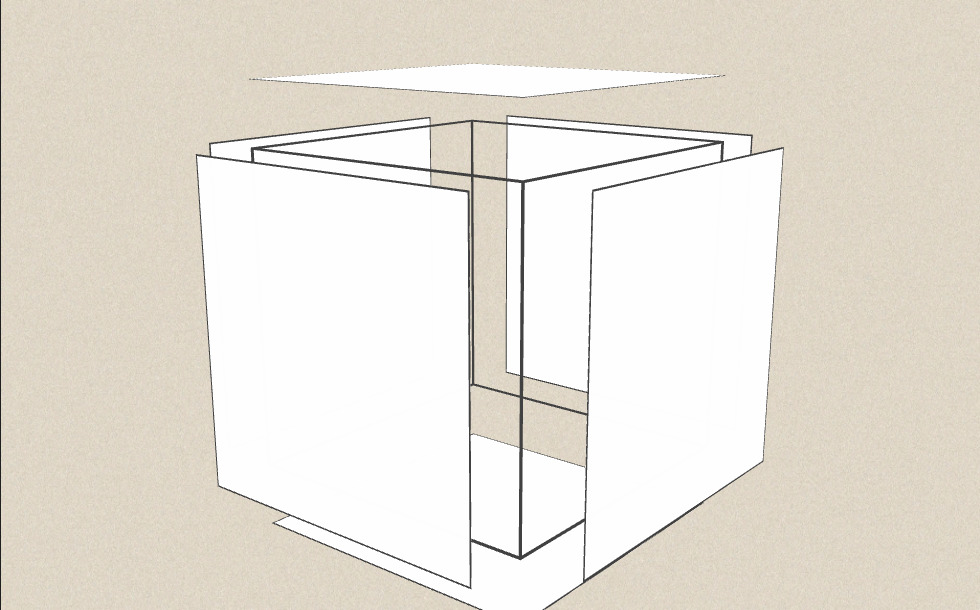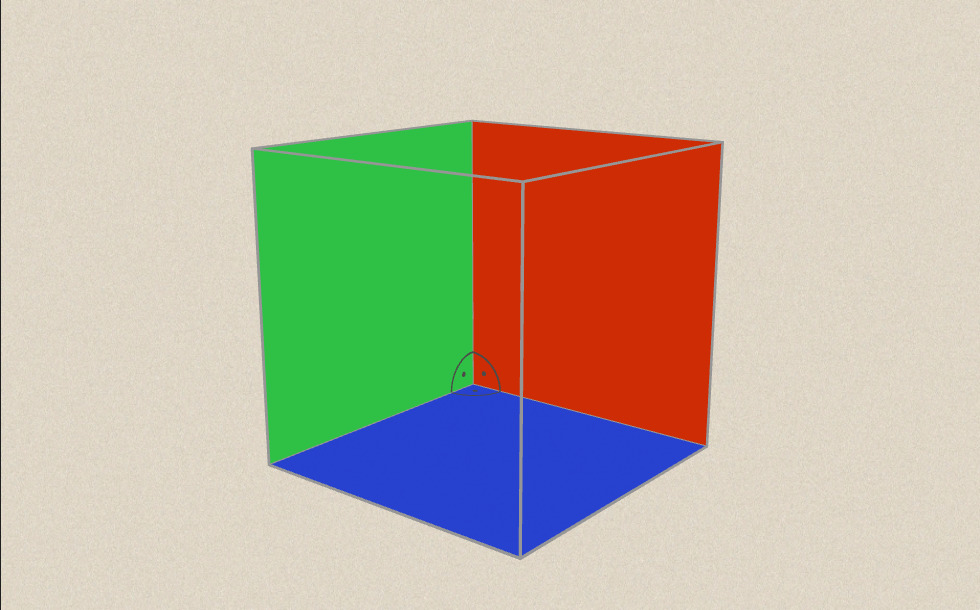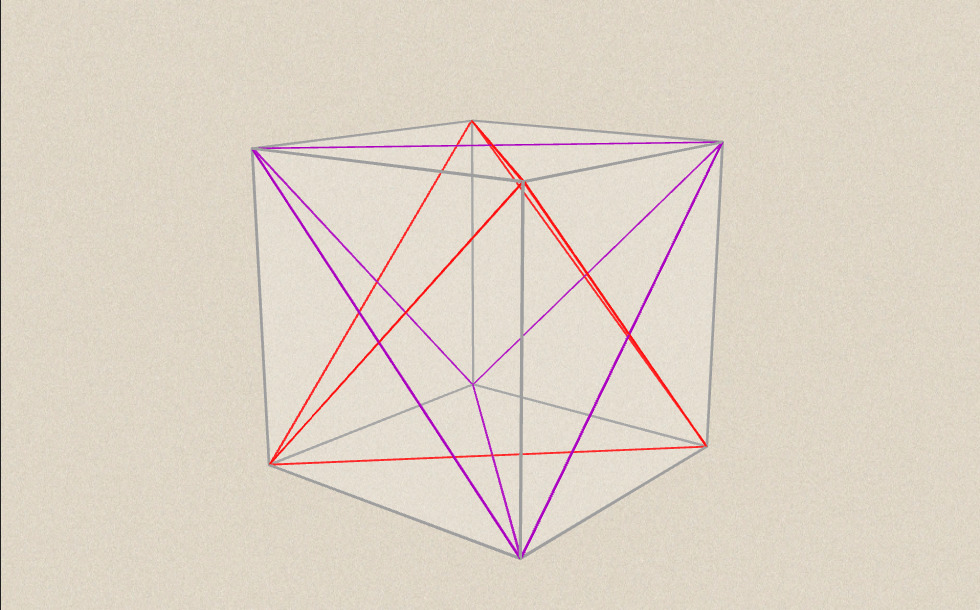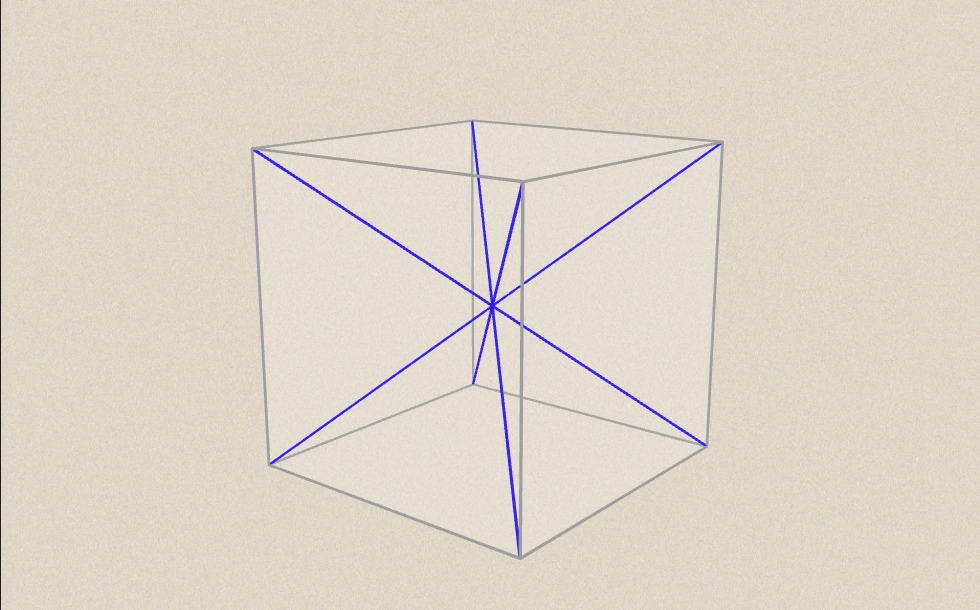### Các mục liên quan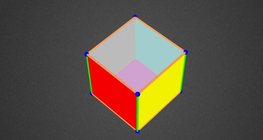#### Colouring a cube

Colouring the vertices, edges and faces of a given cube according to the criteria specified in the exercise.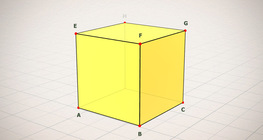#### Cube (exercises)

Edges, diagonals and faces of a cube can be identified by its vertices.#### Cube of cubes

An exercise about the regular hexahedron built from unit cubes to help deepen your knowledge of cubes.#### Net of a cube (exercises)

Not all nets consisting of 6 congruent squares are foldable into cubes.#### Building shapes (one colour)

Build 3D shapes from unit cubes with the help of several views.#### Cube sections (exercise)

Examining solids formed by the intersection of a cube and a plane.#### Cuboid

A cuboid is a polyhedron with six rectangular faces.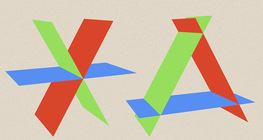#### Dividing Space into Regions by 3 Planes

Division of space by three intersecting planes in several different arrangements.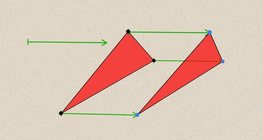#### Geometric transformations – translation

This animation demonstrates geometric translation in both plane and space.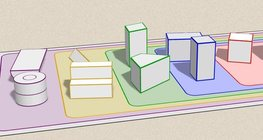#### Grouping of solids

This animation demonstrates various groups of solids through examples.#### Grouping of solids 1

This animation demonstrates various groups of solids through examples.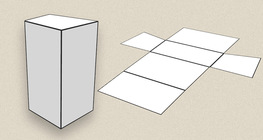#### Lăng trụ

Hoạt cảnh mô tả vài dạng lăng trụ, từ loại chung nhất đến chuyên biệt.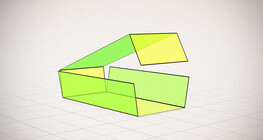#### Nets of a cuboid (exercises)

This animation demonstrates the different nets of a cuboid and includes a game.#### Perimeter, area, surface area and volume

This animation presents the formulas to calculate the perimeter and area of shapes as well as the surface area and volume of solids.#### Platonic solids

This animation demonstrates the five regular three-dimensional (or Platonic) solids, the best known of which is the cube.#### Ratio of volumes of similar solids

This 3D scene explains the correlation between the ratio of similarity and the ratio of volume of geometric solids.#### Volume and surface area (exercise)

An exercise about the volume and surface area of solids generated from a ´base cube´.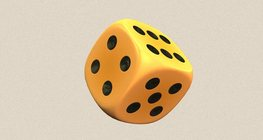#### Dice

Regular dice can be used for solving statistical and probability exercises.